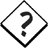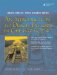# Copy Constructors and Assignment Operators

C++ gives almost god-like powers to the designer of a class. Object "life cycle" management means taking complete control over the behavior of objects during birth, reproduction, and death. We have already seen how constructors manage the birth of an object and how destructors are used to manage the death of an object. This section investigates the reproduction process: the use of copy constructors and assignment operators.

A copy constructor is a constructor that has a prototype like this:

```ClassName(const ClassName & x);
```

The purpose of a copy constructor is to create an object that is an exact copy of an existing object of the same class.

An assignment operator for a class overloads the symbol = and gives it a meaning that is specific to the class. There is one particular version of the assignment operator that has the following prototype:

```ClassName& operator=(const ClassName& x);
```

Because it is possible to have several different overloaded versions of the operator=() in a class, we call this particular version the copy assignment operator (see Example 2.14).

Example 2.14. src/lifecycle/copyassign/fraction.h

 ```[ . . . . ] class Fraction { public: Fraction(int n, int d) : m_Numer(n), m_Denom(d) { ++ctors; } Fraction(const Fraction& other) : m_Numer(other.m_Numer), m_Denom(other.m_Denom) { ++copies; } Fraction& operator=(const Fraction& other) { m_Numer = other.m_Numer; m_Denom = other.m_Denom; ++assigns; return *this; } Fraction multiply(Fraction f2) { return Fraction (m_Numer*f2.m_Numer, m_Denom*f2.m_Denom); } static void report(); private: int m_Numer, m_Denom; static int assigns; static int copies; static int ctors; }; [ . . . . ] ```

The version of Fraction in Example 2.14 defines three static counters, so that we can count the total number of times each member function is called. This should help us better understand when objects are copied. Example 2.15 uses it to create, copy, and assign some objects.

Example 2.15. src/lifecycle/copyassign/copyassign.cpp

 ```#include #include "fraction.h" int main() { using namespace std; Fraction twothirds(2,3); <-- 1 Fraction threequarters(3,4); Fraction acopy(twothirds); <-- 2 Fraction f4 = threequarters; <-- 3 cout << "after declarations" ; Fraction::report(); f4 = twothirds; <-- 4 cout << "before multiply" ; Fraction::report(); f4 = twothirds.multiply(threequarters); <-- 5 cout << "after multiply" ; Fraction::report(); return 0; } ```   (1)using 2-arg constructor (2)using copy constructor (3)also using copy constructor (4)assignment (5)Lots of objects get created here.
```src/ctor> g++ -ansi -pedantic -Wall copyassign.cpp
src/ctor> ./a.out
after declarations
[ assigns: 0 copies: 2 ctors: 2 ]
before multiply
[ assigns: 1 copies: 2 ctors: 2 ]
after multiply
[ assigns: 2 copies: 3 ctors: 3 ]
src/ctor>
```As you can see, the call to multiply creates three Fraction objects. Can you explain why?

It is important to know that the compiler will supply default versions of the copy constructor and/or the copy assignment operator if one or both are missing from the class definition. The compiler-supplied default versions have the following prototypes for a class T:

```T::T(const T& other);
T& T::operator=(const T& other);
```

### ConversionsAn Introduction to Design Patterns in C++ with Qt 4
ISBN: 0131879057
EAN: 2147483647
Year: 2004
Pages: 268